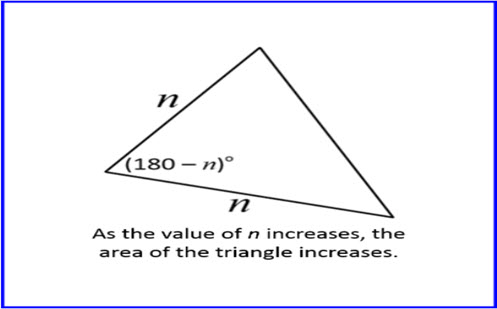Go to website

# Area of a triangle

The prompt will challenge students to think about the area of a non-right-angled triangle, using the general sine formula. They also explore the concept of proof, deepening their conceptual understanding. The resource includes classroom examples, teacher notes and guided questions.

Year level(s) Year 9, Year 10
Audience Teacher
Teaching strategies and pedagogical approaches Collaborative learning, Differentiated teaching, Explicit teaching, Feedback, Mathematics investigation
Keywords sine, triangle, angle, proof, area

## Curriculum alignment

Curriculum connections Critical and creative thinking, Numeracy
Strand and focus Space, Measurement, Algebra, Apply understanding, Build understanding
Topics Algebraic expressions, Angles and geometric reasoning
AC: Mathematics (V9.0) content descriptions
AC9M9SP01
Recognise the constancy of the sine, cosine and tangent ratios for a given angle in right-angled triangles using properties of similarity

AC9M10M03

Solve practical problems applying Pythagoras' theorem and trigonometry of right-angled triangles, including problems involving direction and angles of elevation and depression

Numeracy progression Proportional thinking (P7)
Understanding geometric properties (P7)
Understanding units of measurement (P10)